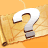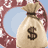# What is the Impact of Destiny Number (Fate Number) on Your LifeNumerology is one of the simplest methods to study an individual and his life path. One can easily predict the nature, life cycle and character of a person through this medium. Likewise, Destiny number or Life Cycle Number is another aspect of numerology. It is also known as Life path number and Individuality number. Through the medium of a destiny number, one can predict the life path of a person including all the important events and can guide him to a positive aspect of life. Calculating a destiny number is as easy as calculating the Root number. Scholars like Kiro and Pythagoras have provided us with the simplification methods to calculate the numbers in Numerology. The procedure to calculate the destiny number is long, but very simple. Destiny number plays an important role in our lives and influences it in many ways. A person and his nature can be known through the medium of Destiny number. Destiny number is used to calculate the important events of life along with their time and date. Even the name change is based on the destiny number. A person is able to know about the ways of protecting himself any kind of mis-happenings and can protect himself accordingly. Thus, it informs us about the many aspects of our lives.

## How to Calculate the Destiny Number

Destiny number is a method to determine the appropriate career options and is considered to be an important aspect of Numerology. Destiny number basically means an important part of your life through which you can determine the career options in your life and can achieve success. It is calculated on the basis of date of birth which includes the month, year and date. For Example : For instance, a person is born on 14th December 1942, then the procedure to calculate the Destiny number is as follows : Date of birth + Month of birth + Year of Birth =  Destiny number Date of birth - 1+ 4 = 5 Month of Birth - 1+ 2 = 1 + 2 = 3 Year of Birth - 1942 = 1+ 9 + 4 + 2 = 16 = 1 + 6 = 7 The destiny number of this person will be 6 ( 5 + 3 + 7 = 15 = 6 ) Date of birth is the base for calculating the Destiny Number as it is the only information that cannot be changed ever about that person and depicts the status of the planets at the time of his birth. To get your Numerology Life Analysis Report, please click on this link : Numerology Life Analysis
Categories: